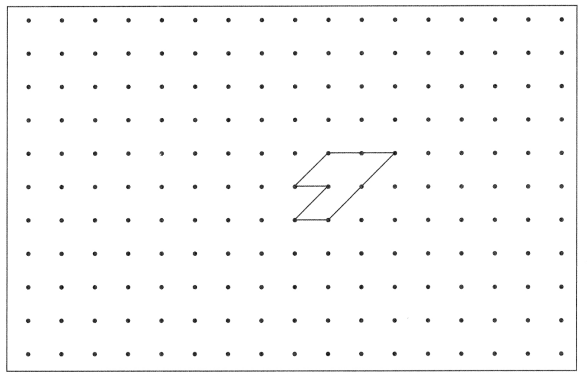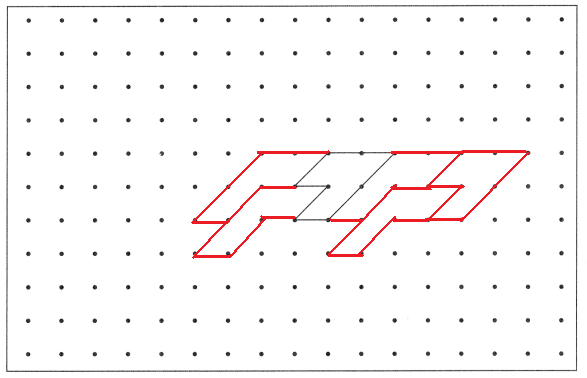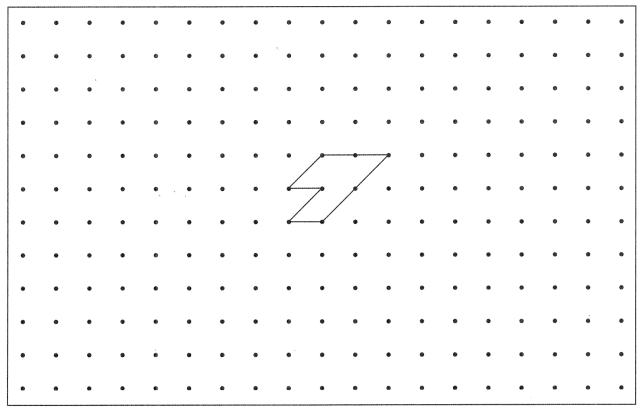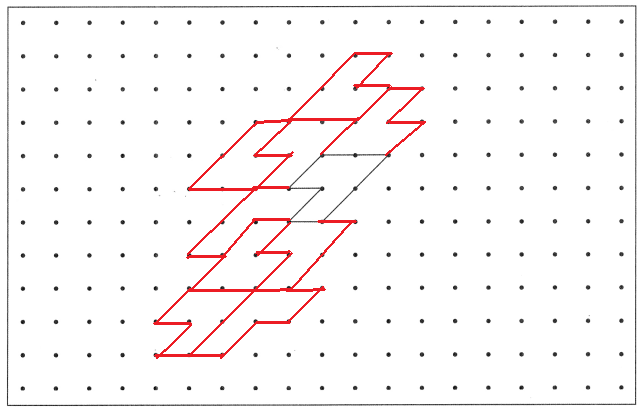# Math in Focus Grade 4 Cumulative Review Chapters 12 to 14 Answer Key

This handy Math in Focus Grade 4 Workbook Answer Key Cumulative Review Chapters 12 to 14 detailed solutions for the textbook questions.

## Math in Focus Grade 4 Cumulative Review Chapters 12 to 14 Answer Key

Concepts and Skills

Estimate the area of each figure. (Lesson 12.1)

Question 1.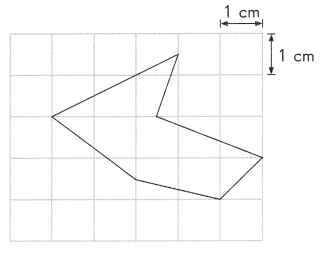Area of a small square is 1 square centimeters.
By seeing the above figure we can estimate the area of the figure is nearly 6 square centimeters.

Question 2.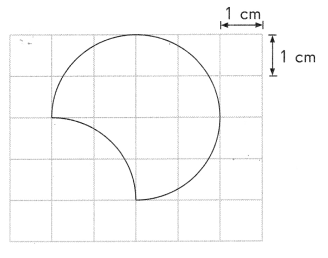Area of a small square is 1 square centimeters.
By seeing the above figure we can estimate the area of the figure is nearly 9 square centimeters.

Solve. Show your work. (Lesson 12.2)

Question 3.
The perimeter of a rectangle is 54 feet. Its length is 14 feet. Find its width.
The perimeter of a rectangle is 54 feet.
Its length is 14 feet.
Width = ?
Perimeter of the rectangle = 2 (l + w)
54 feet = 2 (14 feet + w)
54 feet = 28 feet + 2w
26 feet = 2w
13 feet = w
The width of the rectangle = 13 feet.

Question 4.
The area of a rectangle is 65 square inches. Its width is 5 inches. Find its length.
The area of a rectangle is 65 square inches.
Its width is 5 inches.
Length = ?
Area of the rectangle = l x w
65 square inches = l x 5 inches
13 inches = l
The length of the rectangle is 13 inches.

Is the dotted line in each figure a line of symmetry? Write yes or no. (Lesson 13.1)

Question 5.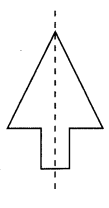Yes, The dotted line in the above figure has a line of symmetry.

Question 6.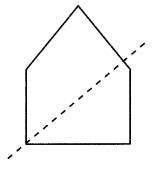No, The dotted line in the above figure doesn’t have a line of symmetry.

Question 7.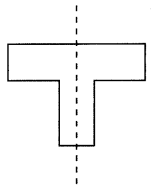Yes, The dotted line in the above figure has a line of symmetry.

Decide whether each figure has rotational symmetry about the center shown. Write yes or no. (Lesson 13.2)

Question 8.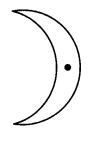Yes, The above figure has rotational symmetry for the center shown in the figure.

Question 9.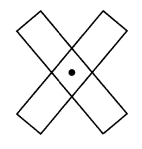Yes, The above figure has rotational symmetry for the center shown in the figure.

Each figure is half of a symmetric shape with the dotted line as its line of symmetry. Complete each symmetric shape. (Lesson 13.3)

Question 10.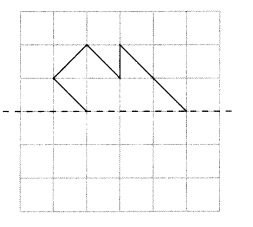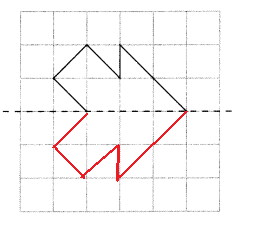The above figure is in symmetric figure.

Question 11.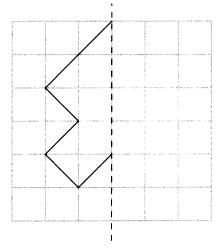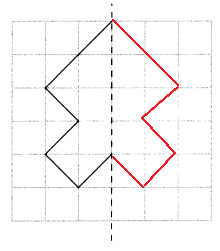The above figure is in symmetric figure.

Each figure is half of a symmetric shape. Complete each symmetric shape so it has rotational symmetry about the center shown. (Lesson 13.3)

Question 12.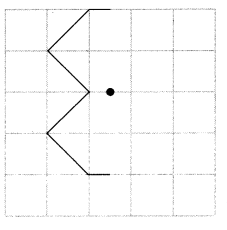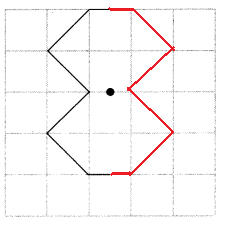Question 13.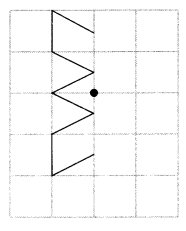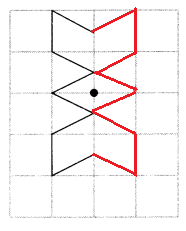Shade the correct squares so that the pattern of shaded squares has rotational symmetry about the given point. (Lesson 13.3)

Question 14.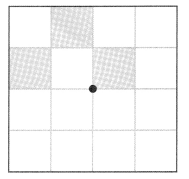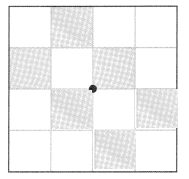Shaded the correct squares. The pattern of shaded squares has rotational symmetry for the given point.

Question 15.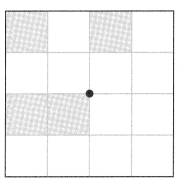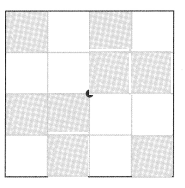Shaded the correct squares. The pattern of shaded squares has rotational symmetry for the given point.

Shade the repeated shape in each tessellation. (Lesson 14.1)

Question 16.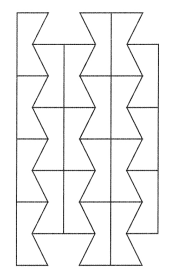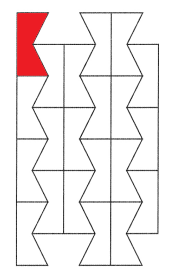Shaded the repeated shape in the above tessellation with red color as we can observe in the above image.

Question 17.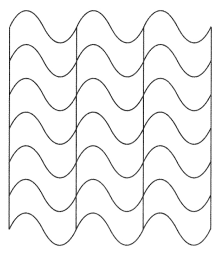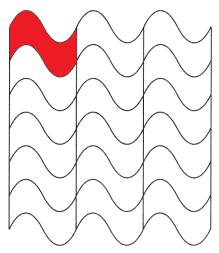Shaded the repeated shape in the above tessellation with red color as we can observe in the above image.

Add nine more repeated shapes to the tessellation. (Lesson 14.1)

Question 18.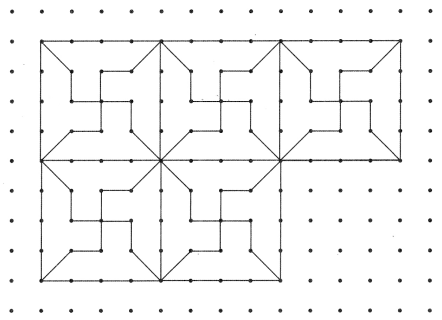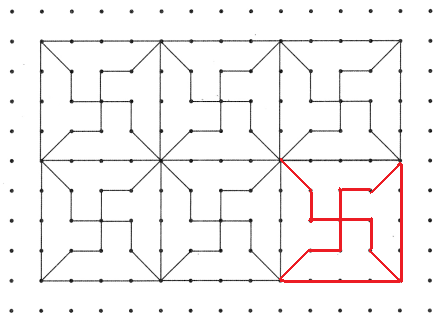Add nine more repeated shapes to the tessellation. (Lesson 14.1)

Question 19.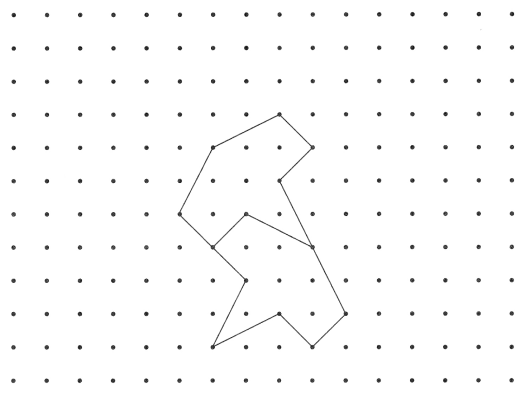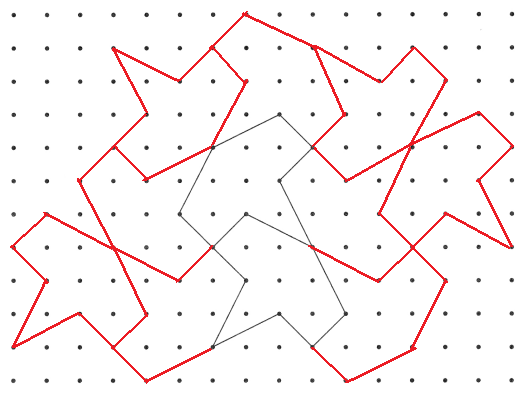Added nine more repeated shapes to the above given tessellation.

Problem Solving

Solve. Show your work. (Lessons 12.3 and 12.4)

Question 20.
This figure is made up of rectangles. Find its perimeter and area.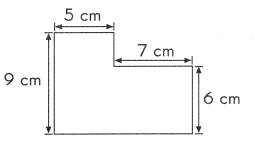Length = 12 cm
width = 9 cm
Perimeter of the rectangle = 2 (l + w)
= 2 (12 + 9)
= 2(21)
= 42 cm
Perimeter of the rectangle is 42 cm.
Area of the rectangle = l x w
= 12 cm x 9 cm
= 108 square centimeters
Area of the rectangle is 108 square centimeters.

Question 21.
A rectangle is divided into 3 identical squares as shown. The area of the rectangle is 1 47 square yards. Find the length and width.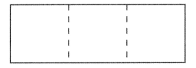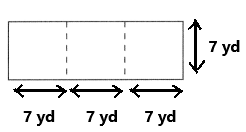The area of the rectangle is 147 square yards.
A rectangle is divided into 3 identical squares as we can observe in the above image.
147/3 = 49 square yards
The area of each square is 49 square yards. The length and width of the square is 7 yards.
Length of the rectangle = 7 yards + 7 yards + 7 yards = 21 yards
Width of the rectangle = 7 yards

Question 22.
A photograph measuring 1 2 centimeters by 9 centimeters is mounted on a rectangular piece of cardboard measuring 20 centimeters by 1 5 centimeters as shown.
Find
a. the area of the border.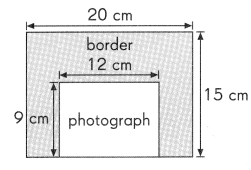b. the perimeter of the border.

Solve. (Lesson 13.1)

Question 23.
In the figure below, do joining points A and B form a line of symmetry? Explain your answer.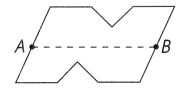No, The joining points A and B doesn’t form a line of symmetry.

Solve. (Lessons 13.1 and 13.2)

Question 24.
Using the letters H, I, M, O, S, and U, form a three-letter symmetrical pattern that has
a. only line symmetry.
HIO forms a three-letter symmetric pattern.

b. only rotational symmetry.
IOS form a three-letter rotational symmetric pattern.

c. both line and rotational symmetry. The letters may be used more than once.
HIO form a three-letter both line and rotational symmetric pattern.

Draw. (Lesson 14.2)

Question 25.
Use the given shape to make two different tessellations,
a. Tessellation 1